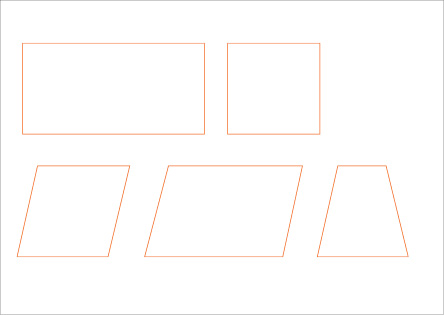# Difference between Congruent and Equal

Unlike many other things, it is difficult for a person to differentiate between congruent and equal until or unless, you have the adequate knowledge that would assist you in stating the differences. You find it hard to identify the difference but nothing is impossible if you struggle for it. In order to state the difference between congruent and equal you must learn it properly. First of all you should understand that unlike the real life, geometrical laws are very strict in such regards. For example, if two persons are of equal height you might call them congruent or same but in geometry this is not the case. If there is similarity in any part of two different things, you can call it equal but if they are similar in terms of the size, magnitude and shape, only then they are called congruent.

If the magnitude or size of any two products is equal, then the products are referred to as equal but if both of these traits are matched with each other, then they are called congruent. The definition of these terms may vary from subject to subject, but mathematically, it says that the some features of both products are same. Regardless of their shape and structure, if they have similarity in a single feature, they are called equal. Equality is generally related in terms of numbers that represent properties. For example, two people having same weight are called equal.

On the other hand, if both pictures have almost everything in common but their lies a difference in just one feature, they will not be called congruent. In terms of the mathematical shapes, if the area of a rectangle and square is same, they will be called equal but in order to be congruent, the area of a square has to be equal to the area of other square, which is similar in shape. Similarly, in terms of a rectangle, it is necessary that both triangles should be identical to each other in terms of magnitude and the angles. Not to mention, these shapes should be able to overlap each other perfectly.

### Instructions

• 1

Congruent

It is a trait of the body or any shape which is identical to the other shape. Congruent is something that has no difference and is equal in magnitude, size, shape and direction.

Image Courtesy: solving-math-problems.com• 2

Equal

If there is similarity in some features of the shapes or objects, they are called equal.

Image Courtesy: schools.nsw.edu.au•